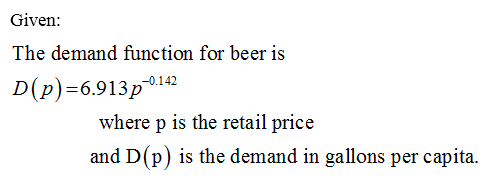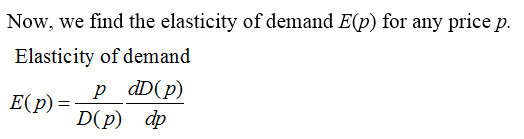# The demand function for beer is given below, where p is the retail price and D(p) is the demand in gallons per capita. For the demand function, find the elasticity of demand E(p) for any price p. [Note: You will find that demand is inelastic. This means that taxation, which acts like a price increase, is an ineffective way of discouraging liquor consumption, but is an effective way of raising revenue.]D(p) = 6.913p−0.142 E(p)=

Question
21 views

The demand function for beer is given below, where p is the retail price and

D(p) is the demand in gallons per capita. For the demand function, find the elasticity of demand E(p) for any price p. [Note: You will find that demand is inelastic. This means that taxation, which acts like a price increase, is an ineffective way of discouraging liquor consumption, but is an effective way of raising revenue.]
D(p) = 6.913p−0.142

E(p)=
check_circle

Step 1Step 2...

### Want to see the full answer?

See Solution

#### Want to see this answer and more?

Solutions are written by subject experts who are available 24/7. Questions are typically answered within 1 hour.*

See Solution
*Response times may vary by subject and question.
Tagged in